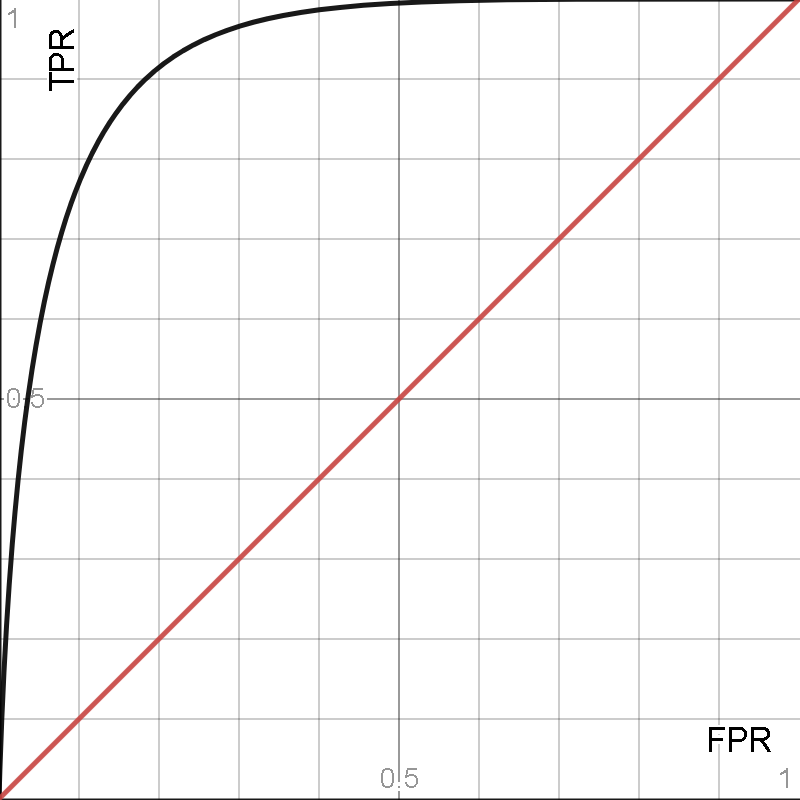Blogs/ROC Curve

# ROC Curve

blazedafyah Aug 26 2021 1 min read 161 views

NIT Warangal graduate, Software Engineer at Wipro.

Fundamentals Prob. and StatsThe Receiver Operating Characteristics (ROC) curve is a performance metric for classification tasks at various classification thresholds.

The ROC curve represents the trade-off between the sensitivity and the specificity of the model.

The ROC curve plots True Positive Rate (TPR) on the y-axis and False Positive Rate (FPR) on the x-axis.

True Positive Rate (TPR) or Sensitivity measures the proportion of positives that are correctly classified i.e. recall and is therefore defined as

TPR = TP/TP+FN

False Positive Rate (FPR) or Specificity measures the proportion of negatives that are correctly classified and is therefore defined as

FPR = TP/TP+FN

The terms TP (true positive), FP (false positive), TN (true negative), and FN (false negative) refer to the result of a test and the correctness of the classification in that order.

# INTERPRETING THE ROC CURVEThe black curve represents the ROC curve of some classifier. The red diagonal indicates the ROC curve of a baseline model which makes random predictions (TPR = FPR). Classifiers whose curves are closer to the top left corner indicate better model performance.

# AUC SCORE

This can be quantified by using the Area Under Curve (AUC) Score. This score can be used to compare the performance of models with respect to each other. The higher the AUC, the better is the model as correctly classifying positives and negatives.

Therefore, a ROC curve gives a good comparative analysis of the correct classifying powers of a classifier. The AUC score also works well as a general measure of classifying accuracy.

Learn and practice this concept here:

https://mlpro.io/problems/roc-curve/